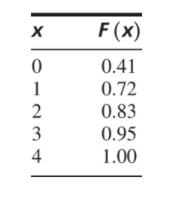×
Get Full Access to Statistics For Engineers And Scientists - 4 Edition - Chapter 2.4 - Problem 8e
Get Full Access to Statistics For Engineers And Scientists - 4 Edition - Chapter 2.4 - Problem 8e

×

# After manufacture, computer disks are tested for errors.ISBN: 9780073401331 38

## Solution for problem 8E Chapter 2.4

Statistics for Engineers and Scientists | 4th Edition

• Textbook Solutions
• 2901 Step-by-step solutions solved by professors and subject experts
• Get 24/7 help from StudySoup virtual teaching assistantsStatistics for Engineers and Scientists | 4th Edition

4 5 1 342 Reviews
16
2
Problem 8E

After manufacture, computer disks are tested for errors. Let X be the number of errors detected on a randomly chosen disk. The following table presents values of the cumulative distribution function F(x) of X.a. What is the probability that two or fewer errors are detected?

b. What is the probability that more than three errors are detected?

c. What is the probability that exactly one error is detected?

d. What is the probability that no errors are detected?

e. What is the most probable number of errors to be detected?

Step-by-Step Solution:

Step 1 of 3

We have computer disks are tested for errors After manufacture. Let us consider the random variable X it presents the number of errors detected on a randomly chosen disk. We have table that  presents values of the cumulative distribution function F(x) of X.

 X F(X) 0 0.41 1 0.72 2 0.83 3 0.95 4 1

Our goal is:

a).We need to find What is the probability that two or fewer errors are detected?

b).We need to find What is the probability that more than three errors are detected?

c).We need to find What is the probability that exactly one error is detected?

d).We need to find  What is the probability that no errors are detected?

e).We need to find  What is the most probable number of errors to be detected?

Step 2 of 3

Step 3 of 3

## Discover and learn what students are asking

Calculus: Early Transcendental Functions : Inverse Trigonometric Functions: Integration
?In Exercises 1-20, find the indefinite integral. $$\int \frac{1}{4+(x-3)^{2}} d x$$

Calculus: Early Transcendental Functions : Moments, Centers of Mass, and Centroids
?Center of Mass of a Two-Dimensional System In Exercises 9-12,find the center of mass of the given system of point masses.

Calculus: Early Transcendental Functions : Partial Derivatives
?In Exercises 7 - 38, find both first partial derivatives. $$z=x^{2} e^{2 y}$$

Statistics: Informed Decisions Using Data : Inference on the Least-Squares Regression Model and Multiple Regression
?First-Year College GPA Researchers at the College Board wanted to build a model that describes one’s first-year college GPA. The researchers obtained

Unlock Textbook Solution